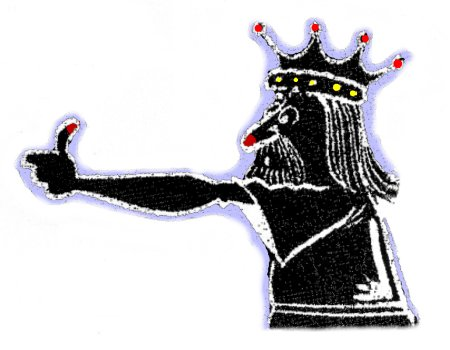# UNITS & MEASUREMENT

`Home`Henry the first's yard.

## Systeme International (S.I.)

The Units System you will be using throughout this Course are Systeme International abbreviated to S.I.

For refrigeration science the following units are the basic units. All others are derived from these:

 Length = metre (m) Time = second (s) Mass = kilogram (kg) Temperature = degree kelvin (K)

Multipliers

Multiplying the basic units by values of 10, or dividing by the same amount enables us to produce a useful series of "shorthand" units which remove the need for cumbersome numbers. For instance, if we have a distance of 10,000 m to work with then we could soon develop large figures with many zero's. However, if we let 1,000 m be called one kilometer (1 km) then our figure of 10,000 m becomes 10 km - much easier to work with.

There are a whole series of these multipliers and sub-multipliers which are used.

The "standard form" of these multipliers puts the multipliers and sub-multipliers into powers of 10.

For instance:

 1 km = 1,000 m 1,000 = 10 x 10 x 10 = 103 1 km = 103 m.

Example

Is there any method of abbreviating the following very cumbersome number yet still keep the units in mm?

5,000,000 [mm]